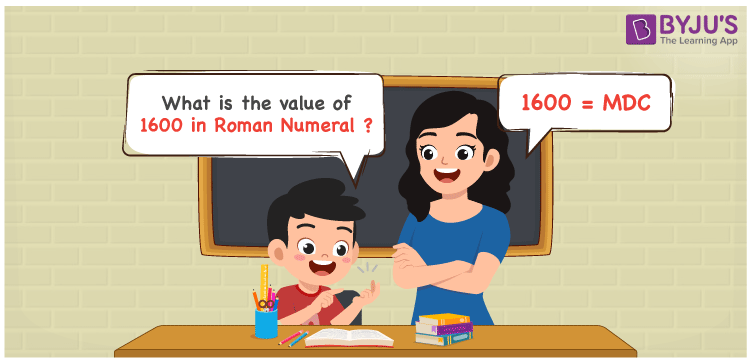# 1600 in Roman Numerals

1600 in Roman Numerals is MDC. Any Roman numeral uses only the 7 primary symbols which are I, V, X, L, C, D, and M, Where M represents 1000, D represents 500 and C represents 100 . What are Roman numerals? can be referred for more details. The details on how to write 1600 are given in the next section of this article.

 Number Roman Numeral 1600 MDC

## How to Write 1600 in Roman Numerals?To convert 1600 in Roman Numerals, we need to represent 1600 as the sum of the fundamental symbols. 1600 can be broken down as the sum of 1000 and 600. Since M is the symbol for 1000, and there is no symbol for 600, it can be written as the sum of 500 and 100. Let us understand it numerically;

1600 = 1000 + 600

1600 = 1000 + 500 + 100

1600 = M + D + C

1600 = MDC.

## Video Lesson on Roman Numerals## Frequently Asked Questions on 1600 in Roman Numerals

Q1

### How to write the number 1600 in Roman Numerals?

1600 in Roman Numerals is written as MDC.
Q2

### Do MDC and CDM represent Roman Numerals?

No. MDC represents Roman Numerals and it is the number 1600, whereas CDM is an invalid Roman numeral.
Q3

### How to express the number One Thousand Six Hundred in Roman numerals?

The number One Thousand Six Hundred is expressed as MDC in Roman Numerals.